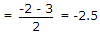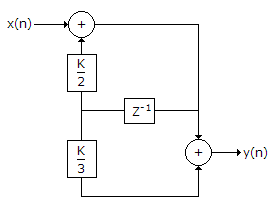# Electronics and Communication Engineering - Exam Questions Papers

16.

An audio signal is band limited to 4 kHz. It is sampled at 8 kHz. What will be the spectrum of the sampled signal?

 A. -4 kHz to 4 kHz B. -8 kHz to 8 kHz C. every 4n kHz repeating D. every ± 8 kHz and operating as well as at zero (k integer)

Explanation:

No answer description available for this question. Let us discuss.

17.

Consider the equation s2 + 2s + 2 + K(s + 2) = 0. Where do the roots of this equation break on the root loci plot?

 A. -3.414 B. -2.414 C. -1.414 D. -0.414

Explanation:

No answer description available for this question. Let us discuss.

18.

The characteristic equation of a closed loop system is (s + 2) (s + 3) + K = 0. The centroid of the asymptotes in root locus is:

 A. 2.5 B. -2.5 C. 0.5 D. -0.5

Explanation:

CentroidPoles are -2 and -3.

19.

Value of 'K' for the system for which the system is stable:A. K < 1 B. K > 1 C. K < 2 D. K > 2

Explanation:

No answer description available for this question. Let us discuss.

20.

The number of roots of s3 + 2s2 + 7s + 3 = 0 in the left half of the s-plane is :

 A. zero B. one C. two D. three